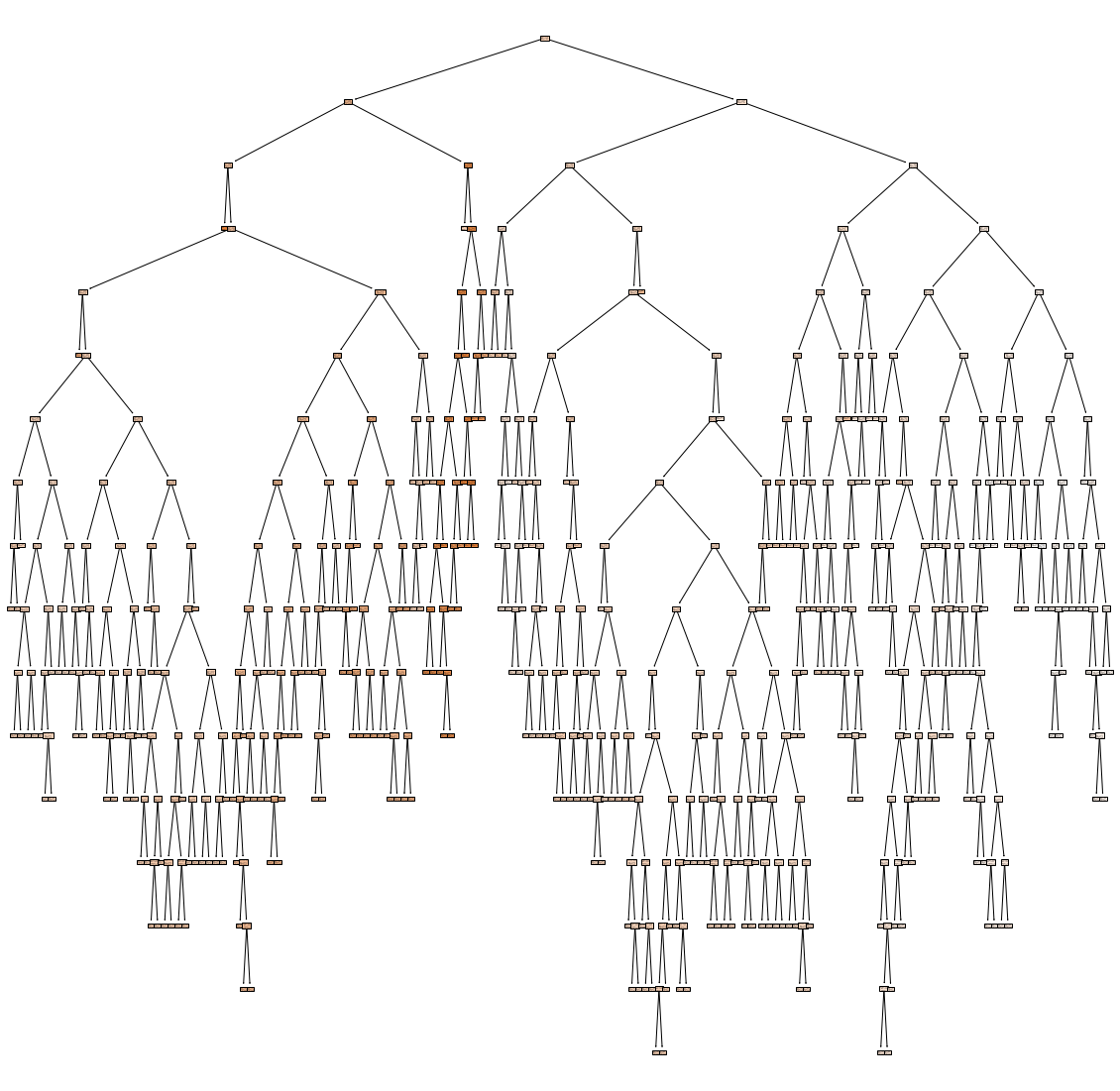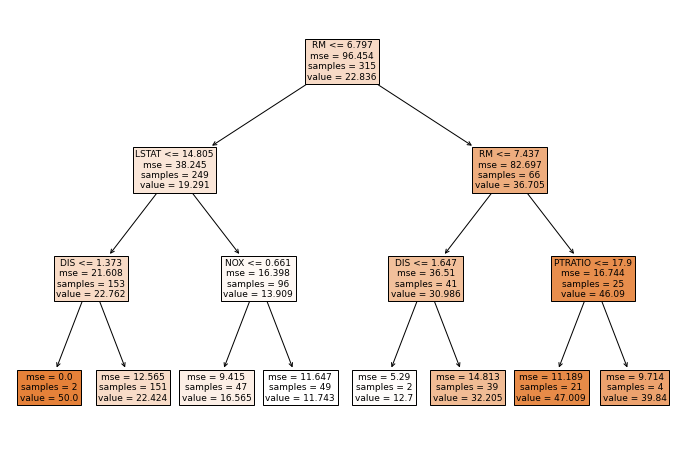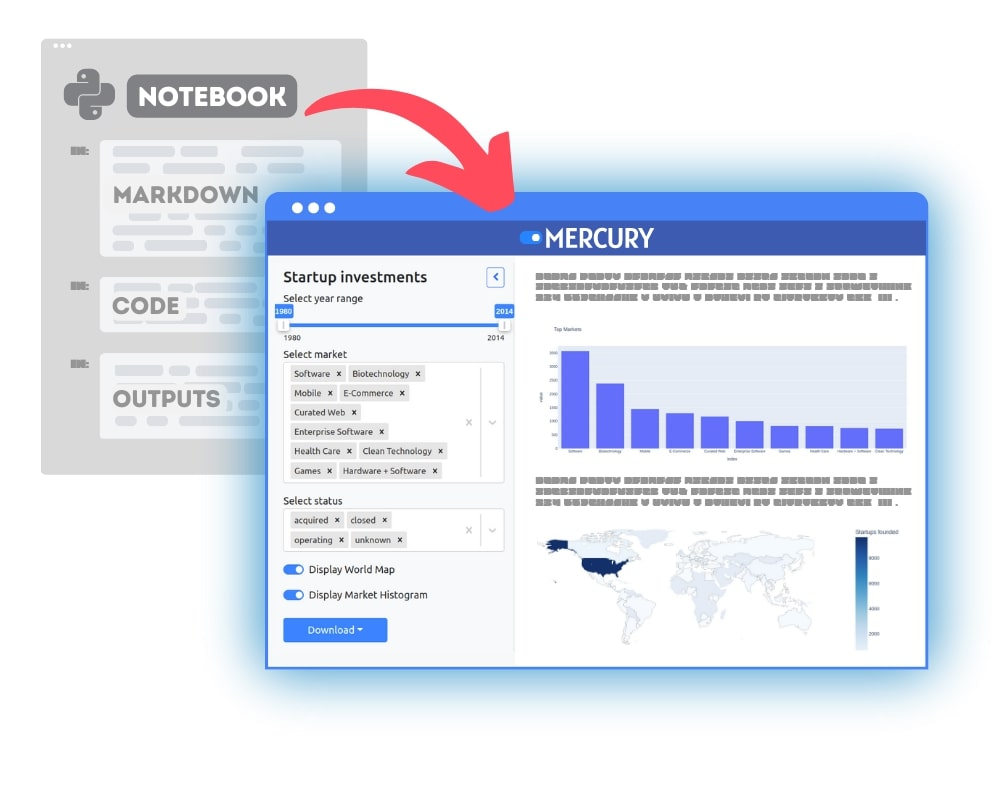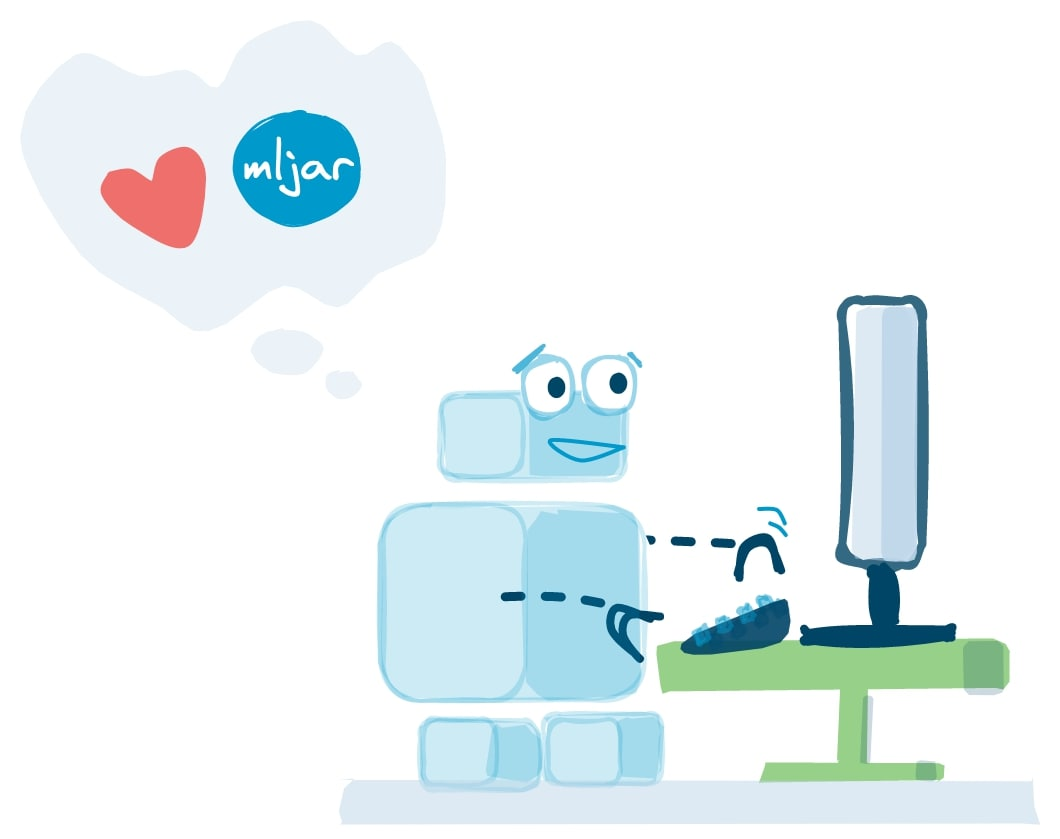The Random Forest is an esemble of Decision Trees. A single Decision Tree can be easily visualized in several different ways. In this post I will show you, how to visualize a Decision Tree from the Random Forest.

First let’s train Random Forest model on Boston data set (it is house price regression task available in `scikit-learn`).

``````# Load packages
import pandas as pd
from sklearn.ensemble import RandomForestRegressor
from sklearn import tree
from dtreeviz.trees import dtreeviz # will be used for tree visualization
from matplotlib import pyplot as plt
plt.rcParams.update({'figure.figsize': (12.0, 8.0)})
plt.rcParams.update({'font.size': 14})
``````

Load the data and train the Random Forest.

``````boston = load_boston()
X = pd.DataFrame(boston.data, columns=boston.feature_names)
y = boston.target
``````

Let’s set the number of trees in the forest to 100 (it is a default of `n_estiamtors`):

``````rf = RandomForestRegressor(n_estimators=100)
rf.fit(X, y)
``````
``````RandomForestRegressor(bootstrap=True, ccp_alpha=0.0, criterion='mse',
max_depth=None, max_features='auto', max_leaf_nodes=None,
max_samples=None, min_impurity_decrease=0.0,
min_impurity_split=None, min_samples_leaf=1,
min_samples_split=2, min_weight_fraction_leaf=0.0,
n_estimators=100, n_jobs=None, oob_score=False,
random_state=None, verbose=0, warm_start=False)
``````

Decision Trees are stored in a `list` in the `estimators_` attribute in the `rf` model. We can check the length of the list, which should be equal to `n_estiamtors` value.

``````len(rf.estimators_)

>>> 100
``````

We can plot a first Decision Tree from the Random Forest (with index `0` in the list):

``````plt.figure(figsize=(20,20))
_ = tree.plot_tree(rf.estimators_, feature_names=X.columns, filled=True)
``````Do you understand anything? The tree is too large to visualize it in one figure and make it readable.

Let’s check the depth of the first tree from the Random Forest:

``````rf.estimators_.tree_.max_depth

>>> 16
``````

Our first tree has `max_depth=16`. Other trees have similar depth. To make visualization readable it will be good to limit the depth of the tree. In MLJAR’s open-source AutoML package mljar-supervised the Decision Tree’s depth is set to be in range from 1 to 4. Let’s train the Random Forest again with `max_depth=3`.

``````rf = RandomForestRegressor(n_estimators=100, max_depth=3)
rf.fit(X, y)
``````
``````RandomForestRegressor(bootstrap=True, ccp_alpha=0.0, criterion='mse',
max_depth=3, max_features='auto', max_leaf_nodes=None,
max_samples=None, min_impurity_decrease=0.0,
min_impurity_split=None, min_samples_leaf=1,
min_samples_split=2, min_weight_fraction_leaf=0.0,
n_estimators=100, n_jobs=None, oob_score=False,
random_state=None, verbose=0, warm_start=False)
``````

The plot of first Decision Tree:

``````_ = tree.plot_tree(rf.estimators_, feature_names=X.columns, filled=True)
``````We can use `dtreeviz` package to visualize the first Decision Tree:

``````viz = dtreeviz(rf.estimators_, X, y, feature_names=X.columns, target_name="Target")
viz
``````## Summary

I show you how to visualize the single Decision Tree from the Random Forest. Trees can be accessed by integer index from `estimators_` list. Sometimes when the tree is too deep, it is worth to limit the depth of the tree with `max_depth` hyper-parameter. What is interesting, limiting the depth of the trees in the Random Forest will make the final model much smaller in terms of used RAM memory and disk space needed to save the model. It will also change the performance of the default Random Forest (with full trees), it will help or not, depending on the data set.### Convert Python Notebooks to Web Apps

We are working on open-source framework Mercury for converting Jupyter Notebooks to interactive Web Applications.### Articles you might find interesing